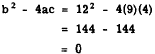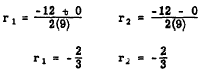Custom SearchEQUAL OR DOUBLE ROOTS If the discriminant b2 - 4ac equals zero, the radical in the quadratic formula becomes zero. In this case the roots are equal; such roots are sometimes called double roots. Consider the equation 9x2 + 12x + 4 = 0 Comparing with the general quadratic, we notice that a = 9, b = 12, and c = 4 The discriminant isTherefore, the roots are equal.  CHECK: From the formulaThe equality of the roots is thus verified. The roots can be equal only if the trinomial is a. perfect square. Its factors are equal. Factoring the trinomial in     9x2 + 12x + 4 = 0 we see that     (3x + 2)2 = 0 Since the factor 3x + 2 is squared, we actually have 3x + 2 = 0 twice, and we havetwice. The fact that the same root must be counted twice explains the use of the term "double root." A double root of a quadratic equation is always rational because a double root can occur only when the radical vanishes. REAL AND UNEQUAL ROOTS When the discriminant is positive, the roots must be real. Also they must be unequal since equal roots occur only when the discriminant is zero. Rational Roots . If the discriminant is a perfect square, the roots are rational. For example, consider the equation 3x2 - x - 2 = 0 in which a = 3, b = -1, and c = -2 The discriminant isWe see that the discriminant, 25, is a perfect square. The perfect square indicates that the radical in the quadratic formula can be removed, that the roots of the equation are rational, and that the trinomial can be factored. In other words, when we evaluate the discriminant and find it to be a perfect square, we know that the trinomial can be factored. Thus,from whichWe see that the information derived from the discriminant is correct. The roots are real, unequal, and rational.